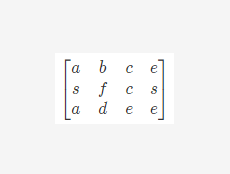矩阵中的路径(Java代码实现附思路)

KLQ 2020-04-13 11:35:14 java常见问答 92580.根据给定数组，初始化一个标志位数组，初始化为false，表示未走过，true表示已经走过，不能走第二次

1.根据行数和列数，遍历数组，先找到一个与str字符串的第一个元素相匹配的矩阵元素，进入judge

2.根据i和j先确定一维数组的位置，因为给定的matrix是一个一维数组

3.确定递归终止条件：越界，当前找到的矩阵值不等于数组对应位置的值，已经走过的，这三类情况，都直接false，说明这条路不通

4.若k，就是待判定的字符串str的索引已经判断到了最后一位，此时说明是匹配成功的

5.下面就是本题的精髓，递归不断地寻找周围四个格子是否符合条件，只要有一个格子符合条件，就继续再找这个符合条件的格子的四周是否存在符合条件的格子，直到k到达末尾或者不满足递归条件就停止。

6.走到这一步，说明本次是不成功的，我们要还原一下标志位数组index处的标志位，进入下一轮的判断。

public class Solution
{
public boolean hasPath(char[] matrix, int rows, int cols, char[] str)
{
//标志位，初始化为false
boolean[] flag = new boolean[matrix.length];
for (int i = 0; i < rows; i++)
{
for (int j = 0; j < cols; j++)
{
//循环遍历二维数组，找到起点等于str第一个元素的值，再递归判断四周是否有符合条件的----回溯法
if (judge(matrix, i, j, rows, cols, flag, str, 0))
{
return true;
}
}
}
return false;
}
//judge(初始矩阵，索引行坐标i，索引纵坐标j，矩阵行数，矩阵列数，待判断的字符串，字符串索引初始为0即先判断字符串的第一位)
private boolean judge(char[] matrix, int i, int j, int rows, int cols, boolean[] flag, char[] str, int k)
{
//先根据i和j计算匹配的第一个元素转为一维数组的位置
int index = i * cols + j;
//递归终止条件
if (i < 0 || j < 0 || i >= rows || j >= cols || matrix[index] != str[k] || flag[index] == true)
return false;
//若k已经到达str末尾了，说明之前的都已经匹配成功了，直接返回true即可
if (k == str.length - 1)
return true;
//要走的第一个位置置为true，表示已经走过了
flag[index] = true;
//回溯，递归寻找，每次找到了就给k加一，找不到，还原
if (judge(matrix, i - 1, j, rows, cols, flag, str, k + 1) ||
judge(matrix, i + 1, j, rows, cols, flag, str, k + 1) ||
judge(matrix, i, j - 1, rows, cols, flag, str, k + 1) ||
judge(matrix, i, j + 1, rows, cols, flag, str, k + 1))
{
return true;
}
//走到这，说明这一条路不通，还原，再试其他的路径
flag[index] = false;
return false;
}
}

/**

*/
public class Solution
{
public boolean hasPath(char[] matrix, int rows, int cols, char[] str)
{
int flag[] = new int[matrix.length];
for (int i = 0; i < rows; i++)
{
for (int j = 0; j < cols; j++)
{
if (helper(matrix, rows, cols, i, j, str, 0, flag))
return true;
}
}
return false;
}
private boolean helper(char[] matrix, int rows, int cols, int i, int j, char[] str, int k, int[] flag)
{
int index = i * cols + j;
if (i < 0 || i >= rows || j < 0 || j >= cols || matrix[index] != str[k] || flag[index] == 1)
return false;
if (k == str.length - 1) return true;
flag[index] = 1;
if (helper(matrix, rows, cols, i - 1, j, str, k + 1, flag) ||
helper(matrix, rows, cols, i + 1, j, str, k + 1, flag) ||
helper(matrix, rows, cols, i, j - 1, str, k + 1, flag) ||
helper(matrix, rows, cols, i, j + 1, str, k + 1, flag))
{
return true;
}
flag[index] = 0;
return false;
}
}

1、每次执行DFS先判断走过的路径是不是等于要求的路径，是就返回结果true，不是就继续找。

2、先判断当前点是否可走，不是，则返回上一层;是就顺时针探寻下一个方位的结点(上、右、下、左)，以下一个方位结点进行DFS，如果方位都探完了还找不到路径，那么当前节点不可能是路径结点之一，只能返回上一层。

public class Solution
{
private boolean visited[] = null; //标志每个位置是否走过，true走过，false没走过
public boolean hasPath(char[] matrix, int rows, int cols, char[] str)
{
if (matrix == null || str == null || str.length > matrix.length || rows * cols != matrix.length)
//进行非法性判断
return false;
//初始化visited数组
visited = new boolean[rows * cols];
for (int i = 0; i < rows; i++)
{
for (int j = 0; j < cols; j++)
{
//以每个结点作为起始节点去dfs
if (dfs(matrix, i, j, rows, cols, str, 0))
return true;
}
}
//没有找到
return false;
}
/**
* @param matrix 地图
* @param x      起点x
* @param y      起点y
* @param rows   行数
* @param cols   列数
* @param str    所求路径
* @param len    当前已经找到路径的长度
* @return
*/
private boolean dfs(char[] matrix, int x, int y, int rows, int cols, char[] str, int len)
{
if (len >= str.length)
{
//找到要求的路径
return true;
}
else
{
//路径长度小于要求的长度，还得继续寻找
if (x >= 0 && x <= rows - 1 && y >= 0 && y <= cols - 1 && visited[x * cols + y] == false && matrix[x * cols + y] == str[len])
{
//当前结点是路径节点之一
visited[x * cols + y] = true; //把当前结点的标志位设为true，证明走过了
//寻找下一方位
int dict = 0;
int x1 = 0, y1 = 0;
while (dict <= 3)
{
switch (dict)
{
case 0:
x1 = x - 1;
y1 = y;
break;
case 1:
x1 = x;
y1 = y + 1;
break;
case 2:
x1 = x + 1;
y1 = y;
break;
case 3:
x1 = x;
y1 = y - 1;
break;
}
if (dfs(matrix, x1, y1, rows, cols, str, len + 1)) //判断下一个方位能否找到路径
return true; //能，直接返回
dict++; //不能，找下一方位
}
//不能从当前结点找不到路径，那就回溯，把当前结点标志位设为false
visited[x * cols + y] = false;
return false; //返回失败
}
else
{
//当前结点不是要找的路径，直接回溯
return false;
}
}
}
}